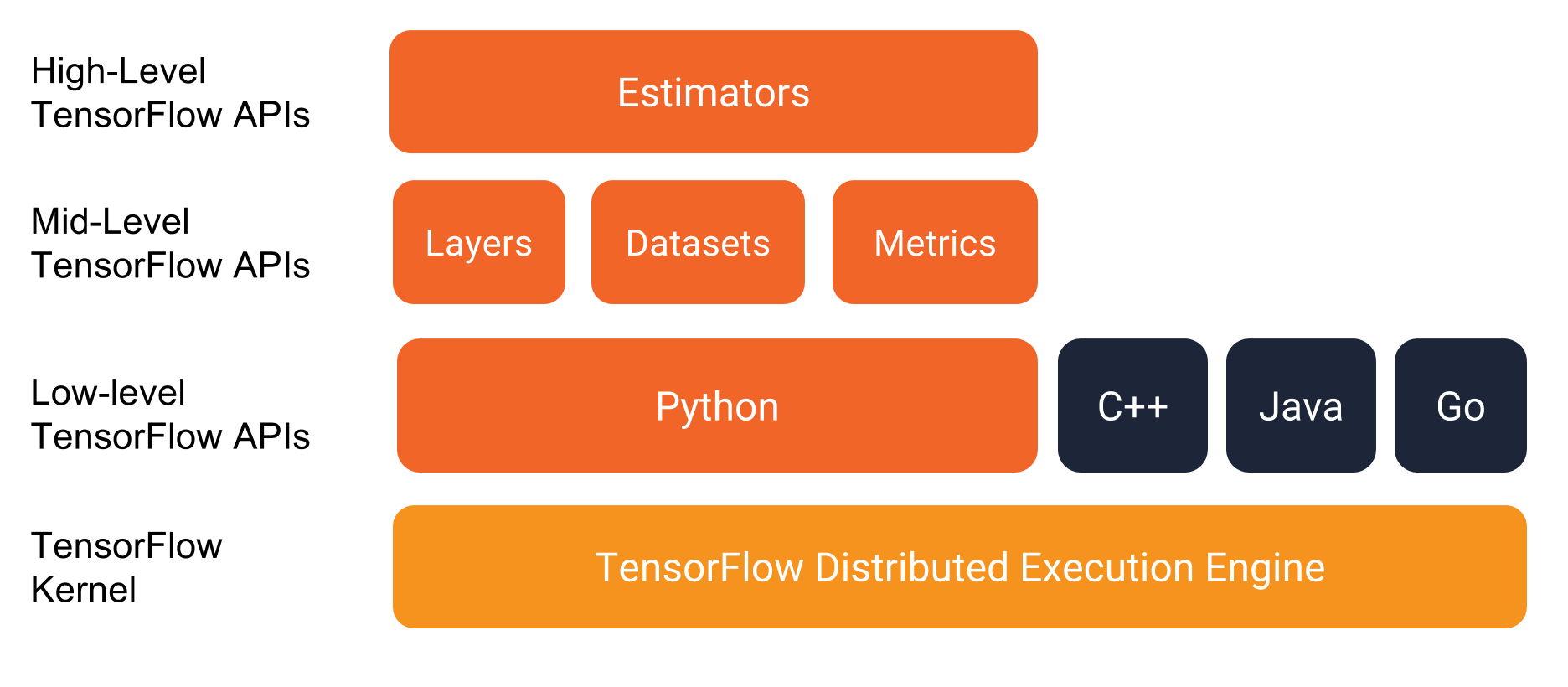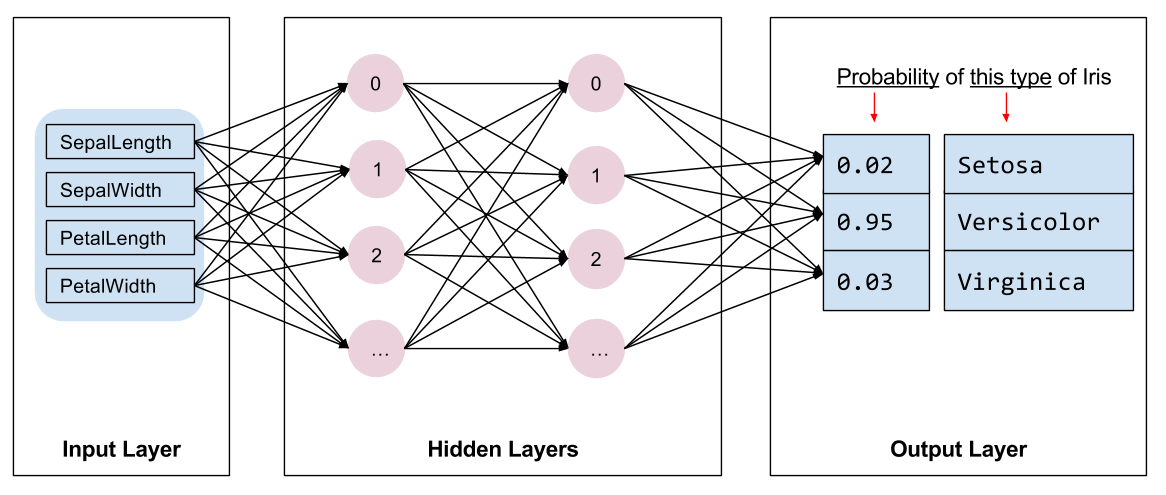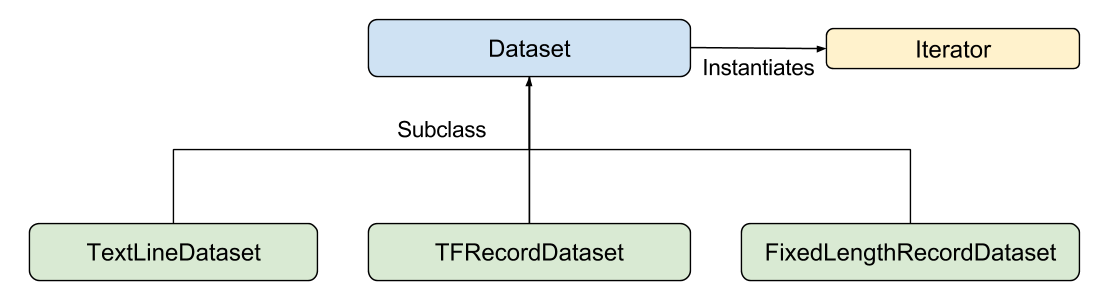## TensorFlow 学习 -- 预创建的 Estimator

858 0

### 快来加入 TensorFlowers 大家庭！

x

• 安装 TensorFlow
• 如果您是使用 virtualenv 或 Anaconda 安装的 TensorFlow，请激活您的 TensorFlow 环境。
• 通过执行以下命令来安装或升级 Pandas：

pip install pandas

• 通过输入以下命令从 GitHub 克隆 TensorFlow 模型代码库：

git clone https://github.com/tensorflow/models
• 将此分支内的目录更改为包含本文档中所用示例的位置：

cd models/samples/core/get_started/

...
Prediction is "Setosa" (99.6%), expected "Setosa"

Prediction is "Versicolor" (99.8%), expected "Versicolor"

Prediction is "Virginica" (97.9%), expected "Virginica"

• 您是否正确安装了 TensorFlow？
• 您使用的 TensorFlow 版本是否正确？
• 您是否激活了 TensorFlow 所在的安装环境？（此问题仅与某些安装机制有关。）• Estimator：代表一个完整的模型。Estimator API 提供一些方法来训练模型、判断模型的准确率并生成预测。
• 数据集：构建数据输入管道。Dataset API 提供一些方法来加载和操作数据，并将数据馈送到您的模型中。Dataset API 与 Estimator API 合作无间。• 花萼长度
• 花萼宽度
• 花瓣长度
• 花瓣宽度

• 山鸢尾 (0)
• 变色鸢尾 (1)
• 维吉尼亚鸢尾 (2)

5.1
3.3
1.7
0.5
0（山鸢尾）

5.0
2.3
3.3
1.0
1（变色鸢尾）

6.4
2.8
5.6
2.2
2（维吉尼亚鸢尾）

• 2 个隐藏层。
• 每个隐藏层包含 10 个节点。• 0.03（山鸢尾）
• 0.95（变色鸢尾）
• 0.02（维吉尼亚鸢尾）

Estimator 是 TensorFlow 对完整模型的高级表示。它会处理初始化、日志记录、保存和恢复等细节部分，并具有很多其他功能，以便您可以专注于模型。有关更多详情，请参阅 Estimator
Estimator 是从 tf.estimator.Estimator 衍生而来的任何类。TensorFlow 提供一组预创建的 Estimator（例如 LinearRegressor）来实现常见的机器学习算法。除此之外，您可以编写自己的自定义 Estimator。我们建议在刚开始使用 TensorFlow 时使用预创建的 Estimator。在掌握预创建的 Estimator 的专业知识之后，我们建议通过创建自己的自定义 Estimator 来优化模型。

• 创建一个或多个输入函数。
• 定义模型的特征列。
• 实例化 Estimator，指定特征列和各种超参数。
• 在 Estimator 对象上调用一个或多个方法，传递适当的输入函数作为数据的来源。

• features - Python 字典，其中：
• 每个键都是特征的名称。
• 每个值都是包含此特征所有值的数组。
• label - 包含每个样本的标签值的数组。

def input_evaluation_set():
features = {'SepalLength': np.array([6.4, 5.0]),
'SepalWidth':  np.array([2.8, 2.3]),
'PetalLength': np.array([5.6, 3.3]),
'PetalWidth':  np.array([2.2, 1.0])}
labels = np.array([2, 1])
return features, labels• Dataset - 包含创建和转换数据集的方法的基类。您还可以通过该类从内存中的数据或 Python 生成器初始化数据集。
• TextLineDataset - 从文本文件中读取行。
• TFRecordDataset - 从 TFRecord 文件中读取记录。
• FixedLengthRecordDataset - 从二进制文件中读取具有固定大小的记录。
• Iterator - 提供一次访问一个数据集元素的方法。
Dataset API 可以为您处理很多常见情况。例如，使用 Dataset API，您可以轻松地从大量并行文件中读取记录，并将它们合并为单个数据流。

def train_input_fn(features, labels, batch_size):
"""An input function for training"""
# Convert the inputs to a Dataset.
dataset = tf.data.Dataset.from_tensor_slices((dict(features), labels))

# Shuffle, repeat, and batch the examples.
return dataset.shuffle(1000).repeat().batch(batch_size)

# Feature columns describe how to use the input.
my_feature_columns = []
for key in train_x.keys():
my_feature_columns.append(tf.feature_column.numeric_column(key=key))

# Build a DNN with 2 hidden layers and 10 nodes in each hidden layer.
classifier = tf.estimator.DNNClassifier(
feature_columns=my_feature_columns,
# Two hidden layers of 10 nodes each.
hidden_units=[10, 10],
# The model must choose between 3 classes.
n_classes=3)

• 训练模型。
• 评估经过训练的模型。
• 使用经过训练的模型进行预测。

# Train the Model.
classifier.train(
input_fn=lambda:iris_data.train_input_fn(train_x, train_y, args.batch_size),
steps=args.train_steps)

# Evaluate the model.
eval_result = classifier.evaluate(
input_fn=lambda:iris_data.eval_input_fn(test_x, test_y, args.batch_size))

print('\nTest set accuracy: {accuracy:0.3f}\n'.format(**eval_result))

Test set accuracy: 0.967

# Generate predictions from the model
expected = ['Setosa', 'Versicolor', 'Virginica']
predict_x = {
'SepalLength': [5.1, 5.9, 6.9],
'SepalWidth': [3.3, 3.0, 3.1],
'PetalLength': [1.7, 4.2, 5.4],
'PetalWidth': [0.5, 1.5, 2.1],
}

predictions = classifier.predict(
input_fn=lambda:iris_data.eval_input_fn(predict_x,
batch_size=args.batch_size))
predict 方法返回一个 Python 可迭代对象，为每个样本生成一个预测结果字典。以下代码输出了一些预测及其概率：

template = ('\nPrediction is "{}" ({:.1f}%), expected "{}"')

for pred_dict, expec in zip(predictions, expected):
class_id = pred_dict['class_ids']
probability = pred_dict['probabilities'][class_id]

print(template.format(iris_data.SPECIES[class_id],
100 * probability, expec))

...
Prediction is "Setosa" (99.6%), expected "Setosa"

Prediction is "Versicolor" (99.8%), expected "Versicolor"

Prediction is "Virginica" (97.9%), expected "Virginica"本版积分规则 回帖后跳转到最后一页